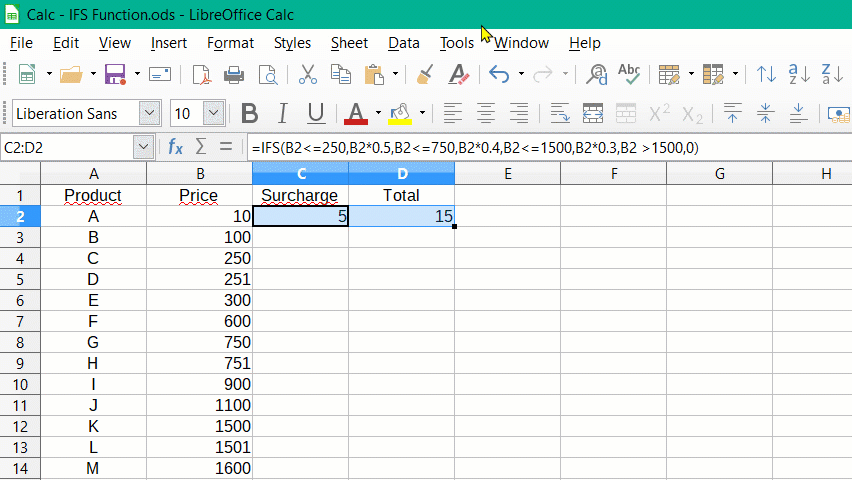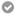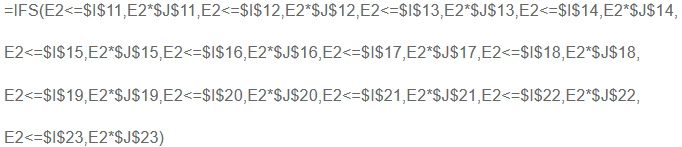# Calculate Ranges - staggered calculation

I have a huge list of products with a wide range of prices. Is there a formula or other way to calculate a markup based on a range? For example, if price range is equal to \$1 to \$250: 50%, \$251 to \$750: 40%, \$751 to \$1,500: 30% and so on. I’m not sure how to search for this if its even possible. I know how to calculate the markup percentage, multiply the price x 1.50, 1.40, 1.30 etc but I’m not sure if it can be done with a price range.

You can have simpler formulas through the use of an auxiliary table and function VLOOKUP().

In a small area, you enter your markup data as 250, 50% in adjacent cells, 750, 40% in next row, … and add a last “guard row” 99999, 0%. Say this table is at Y1:Z10.

When you need a markup value, you compute `VLOOKUP(*product_price*; Y1:Z10; 2; TRUE)` which will return the percentage entered in the second column. See built-in help for paramerter details. The last parameter TRUE tells the first column is sorted in ascending order, allowing to retrieve markup for intermediate values of price.

Give a name to the table instead of Y1:Z10, e.g. Surcharge, so that the formula for the markup readds `VLOOKUP(*product_price*; Surcharge; 2; TRUE)`. Now when you want to change your markups, you only need to update the table without modifying the formulas. Also, you can extend the table (don’t forget to also update the “Surcharge” name range) without having to care for your formulas.

To show the community your question has been answered, click the ✓ next to the correct answer, and “upvote” by clicking on the ^ arrow of any helpful answers. These are the mechanisms for communicating the quality of the Q&A on this site. Thanks!

1 Like

I’ve essentially done this by pointing the formula to a set of cells. I don’t have to modify the formula since its pointing to cells that I can directly modify. I can change the values in the cells and it will change my entire price list for those price ranges. With over 4300 prices, I need it to be fast. I feel VLOOKUP is a lot of extra steps to do something I need to have done quickly so I can get my products re-imported into my software.

Not at all. I routinely use VLOOKUP() when I want to choose a apply a “linear-by-segment” function. It comes in very handy, results in a very compact form and avoids the lengthy IFS() expression when only a factor is different between the “Testn” conditions.

Performance-wise, it is faster than IFS() because you don’t compute repeatedly the Testn until one is true. Since the values in the first column are sorted, Calc applies a very clever search algorithm. You have 4300+ prices but certainly at most 10 markup values. Then finding one is such a small table is fast. After that, you have anyway to compute 4300+ final balues but with a very simple formula (the same as any of yours but for the VLOOKUP() call). The cost in your case comes from decoding the various Testn and computing the logical value by interpretation.

Hi ajlittoz
Apologies if I’m bringing up an old post. I had been searching around for exactly this kind of calculated range formula. I’m wondering if something similar to this can be done in the tables of LibreOffice Writer? I know that some calculations can be done, but is is more limited, and the syntax is bit different. I ask because I’ve already created multiple forms for my company in writer, using tables. It would be awesome if I could just input a formula into what I’ve already made.
This may not be possible, but I figured it wouldn’t hurt to ask.

No, Writer tables don’t have such functions.

I think you can do it with the IFS function. The function can be expanded as desired.

You only have to set up the function once, e.g. in cell C2.

Then you can just pull it down.Formula View:

I wish you success!

If my answer has solved your problem, please click on the checkmark ✓ in the circleto the left of the answer and click on the arrow ^ for upvote. This will tell the community that the question has been answered correctly.

With me Windows 10 Home; Version 1903; 64-Bit | LibreOffice, Version: 6.2.5.2 (x64).

Thank you for the info. this worked perfectly. In fact, I took it a step further by putting the values to be calculated in their own cells.

This gives me the ability to change the ranges on the fly without having to drag the formula down the entire sheet.

I don’t have enough points to upvote.

I have Column A and B as my SKU and Description. Column C, D, E are my Retail Price, List Price and Cost Price.

I’ve added the values I want to use for the calculation in Column I and J.

I’ve pointed the formula to these columns.

I then locked the formula to those cells. Here is the final formula I’m using:Very well!

I gave you a little karma to vote.

Awesome!! This formula works exactly how I need it! Thank you so much for showing me this!! I’ve upvoted your answer.

Thanks so much!